# GSEB Solutions Class 7 Maths Chapter 11 Perimeter and Area Ex 11.4

Gujarat Board GSEB Textbook Solutions Class 7 Maths Chapter 11 Perimeter and Area Ex 11.4 Textbook Questions and Answers.

## Gujarat Board Textbook Solutions Class 7 Maths Chapter 11 Perimeter and Area Ex 11.4Question 1.
A garden is 90 m long and 75 m broad. A path 5 m wide is to be built outside and around it. Find the area of the path. Also find the area of the garden in hectare.
Solution:
Length of the garden (l) = 90 m
Breadth of the garden (b) = 75 mSince, the garden along-with the path makes the outer rectangle.
∴ Length of the outer rectangle
= (90 + 5 + 5) m = 100 m
= (75 + 5 + 5) m = 85 m
∴ Area of the outer path = 100 m x 85 m = 8500 m²
Now, area of the path = [Area of outer rectangle] – [Area of the inner rectangle]
= 8500 m² – 6750 m² = 1750 m².Question 2.
A 3 m wide pathuns outside and around a rectangular park of length 125 m and breadth 65 m. Find the area of the path.
Solution:
Area of the inner region = 125 m x 65 m = 8125 m²
Length of outer region = (125 + 3 + 3)m = 131m
Breadth of the outer region = (65 + 3 + 3) m = 71 mArea of the outer region
= (131 x 71) m² = 9301 m²
Now, Area of the path
= Area of the shaded region
= [Area of the outer region] – [Area of the inner region]
= 9301 m² – 8125 m² = 1176 m²

Question 3.
A picture is painted on a cardboard 8 cm long and 5 cm wide such that there is a margin of 1.5 cm along each of its sides. Find the total area of the margin.
Solution:
Here,
Length of the outer rectangle = 8 cm
Breadth of the outer rectangle = 5 cm
∴ Area of the outer rectangle
= 8 x 5 cm²
= 40 cm²
Width of the margin = 1.5 cm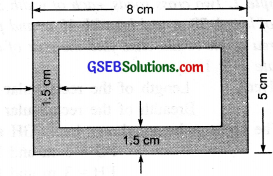∴ For the inner rectangle,
Length = (8 cm – 1.5 cm – 1.5 cm) = 5 cm
Breadth = (5 cm – 1.5 cm – 1.5 cm) = 2 cm
∴ Area of inner rectangle = 5 x 2 cm² = 10 cm²
∴ Area of the margin = [Area of the outer rectangle] – [Area of the inner rectangle]
= 40 cm² – 10 cm² = 30 cm²Question 4.
A verandah of width 2.25 m is constructed all along outside a room which is 5.5 m long and 4 m wide. Find:
(i) The area of the verandah.
(ii) The cost of cementing the floor of the verandah at the rate of ₹ 200 per m².
Solution:
(i) Length of the room = 5.5 m
Breadth of the room = 4 m
Area of the room = 5.5 m x 4 m = 22 m²
Now, width of the verandah = 2.25 mFor the outer rectangle:
Length = [5.5 + 2.25 + 2.25] m = 10 m
Breadth = [4 + 2.25 + 2.25] m = 8.5 m
∴ Area of the outer rectangle = 10 x 8.5 m² = 85 m²
So, area of the verandah = [Area of the outer rectangle] – [Area of the inner rectangle]
= 85 m²- 22 m² = 63 m²

(ii) Rate of cementing = ₹ 200/m²
∴ Cost of cementing the floor of the verandah = ₹ 200 x 63 = ₹ 12,600.
Thus, the required cost of cementing the floor of the verandah is ₹ 12,600.Question 5.
A path 1 m wide is built along the border and inside a square garden of side 30 m. Find:
(i) The area of the path.
(ii) The cost of planting grass in the remaining portion of the garden at the rate of ₹ 40 per m²
Solution:
(i)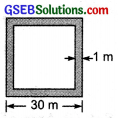Length of the outer square = 30 m
∴ Area of the outer square = 30 m x 30 m = 900 m²
∵ Width of the path = 1 m
∴ Side of the inner square = [30 – 1 – 1] m = 28 m
∴ Area of the inner square = (28 x 28) m² = 784 m²
∴ Area of the path = [Area of the outer square] – [Area of the inner square]
= 900 m² – 784 m² = 116 m²

(ii) ∵ Rate of planting grass = ₹ 40/m².
∴ Cost of planting grass in the remaining portion = ₹ 40 x 784 = ₹ 31,360.

Question 6.
Two cross roads, each of width 10 m, cut at right angles through the centre of a rectangular park of length 700 m and breadth 300 m and parallel to its sides. Find the area of the roads. Also find the area of the park excluding cross roads. Give the answer in hectares.
Solution:
Length of the rectangular park = 700 m
Breadth of the rectangular park = 300 m
Width of the road = 10 m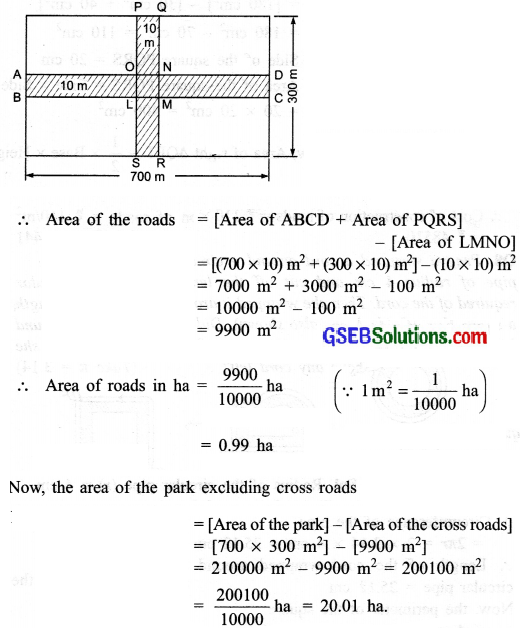Question 7.
Through a rectangular field of length 90 m and breadth 60 m, two roads are constructed which are parallel to the sides and cut each other at right angles through the centre of the fields. If the width of each road is 3 m, find:
(i) the area covered by the roads.
(ii) the cost of constructing the roads at the rate of ₹ 110 per m².
Solution:
Length of the rectangular field = 90 m
Breadth of the rectangular field = 60 m
Width of each road = 3 m
∴ Area of ABCD = 90 x 3 m² = 270 m²
Area of EFGH = 60 x 3 m² = 180 m²
Area of PQRS = 3 x 3 m² = 9 m²(i) ∴ Area of roads = [(Area of ABCD) + (Area of EFGH)] – [Area of PQRS]
= [270 m² + 180 m²] – 9 m²
= 450 m² – 9 m² = 441 m²

(ii) Rate of construction of roads = ₹ 110/m²
∴ Cost of construction of roads = ₹ 110 x 441 = ₹ 48510Question 8.
Pragya wrapped a cord around a circular pipe of radius 4 cm and cut off the length required of the cord: Then she wrapped it around a square box of side 4 cm (also shown). Did she have any cord left? (Take π = 3.14)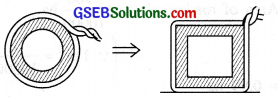Solution:
Radius of the circular pipe (r) = 4 cm
∴ Circumference of the pipe = 2πr = 2 x 3.14 x 4 cm = 25.12 cm
∴ Length of the cord wrapped around the circular pipe = 25.12 cm
Now, the perimeter of the square = 4 x Side = 4 x 4 cm = 16 cm
∴ Length of the cord wrapped around the square = 16 cm
Since 25.12 cm > 16 cm and (25.12 – 16) cm = 9.12 cm
Thus, we can say, yes, the cord (9.12 cm) is left with Pragya.

Question 9.
The adjoining figure represents a rectangular lawn with a circular flower bed in the middle. Find: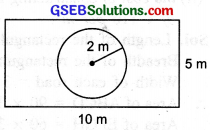(i) the area of the whole land.
(ii) the area of the flower bed.
(iii) the area of the lawn excluding the area of the flower bed.
(iv) the circumference of the flower bed.
Solution:
Length of the plot = 10 m
Breadth of the plot = 5 m
(i) ∴ Area of the plot (whole land) 5 m = 50 m²

(ii) Radius of the circular flower bed (r) = 2 m
∴ Area of the flower bed = πr²
= 3.14 x 2 x 2 m²
= 12.56 m²

(iii) ∴ Area of the lawn excluding the area of the flower bed = 50 m² – 12.56 m² = 37.44 m²

(iv) Circumference of the circular flower bed = 2πr
= 2 x 3.14 x 2 m = 12.56 mQuestion 10.
In the following figures, find the area of the shaded portions: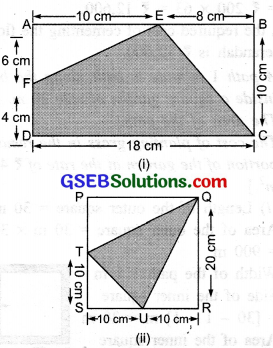Solution:
(i) Area of the whole rectangle ABCD
= 18 x 10 cm = 180 cm²
Area of right ∆AEF = $$\frac { 1 }{ 2 }$$ x Base x Height = $$\frac { 1 }{ 2 }$$ x 6 x 10 cm = 30 cm²
Area of right ∆CBE = $$\frac { 1 }{ 2 }$$ x Base x Height = $$\frac { 1 }{ 2 }$$ x 8 x 10 cm²
∴ Area of the shaded portion = [Area of ABCD] – [Area of ∆AEF + Area of ∆CBE]
= [180 cm²] – [30 cm² + 40 cm²]
= 180 cm² – 70 cm² = 110 cm²

(ii) Side of the square PQRS = 20 cm
∴ Area of the square PQRS = Side x Side
= 20 x 20 cm² = 400 cm²
Now, Area of right ∆QPT = $$\frac { 1 }{ 2 }$$ x Base x Height
= $$\frac { 1 }{ 2 }$$ x 20 cm x 10 cm = 100 cm²
Area of right ∆TSU = $$\frac { 1 }{ 2 }$$ x Base x Height
= $$\frac { 1 }{ 2 }$$ x 10 x 10 cm² = 50 cm²
Area of right ∆QRU = $$\frac { 1 }{ 2 }$$ x Base x Height
= $$\frac { 1 }{ 2 }$$ x 10 x 20 cm² = 100 cm²
∴ Area of the shaded portion = [Area PQRS] – [Area of ∆QPT + Area of ∆TSU + Area of ∆QRU]
= [400 cm²] – [100 cm² + 50 cm² + 100 cm²]
= 400 cm² – 250 cm² = 150 cm²Question 11.
Find the area of the quadrilateral ABCD. Here, AC = 22 cm, BM = 3 cm, DN = 3 cm, and BM ⊥ AC, DN ⊥ AC.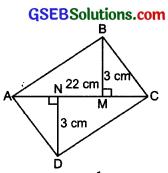Solution:
Area of ∆ABC = $$\frac { 1 }{ 2 }$$ x AC x [Altitude corresponding to AC]
= $$\frac { 1 }{ 2 }$$ x 22 x 3 cm² = 33 cm²
Area of ∆ACD =$$\frac { 1 }{ 2 }$$ x AC x [Altitude corresponding to AC]
= $$\frac { 1 }{ 2 }$$ x 22 x 3 cm²
= 33 cm²